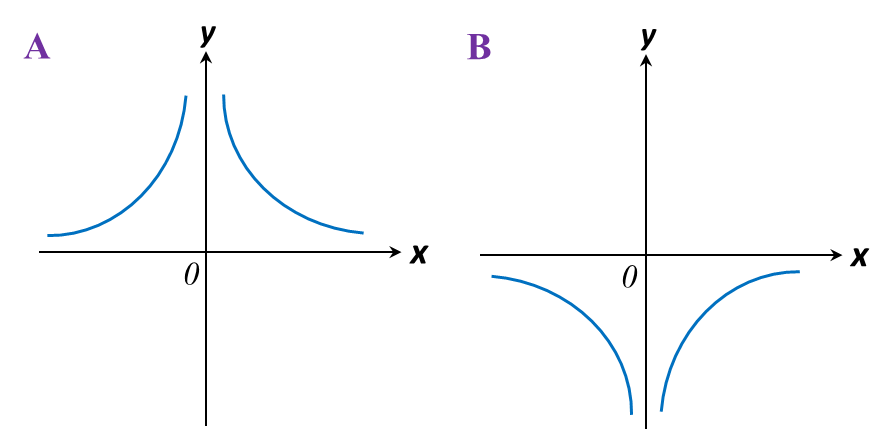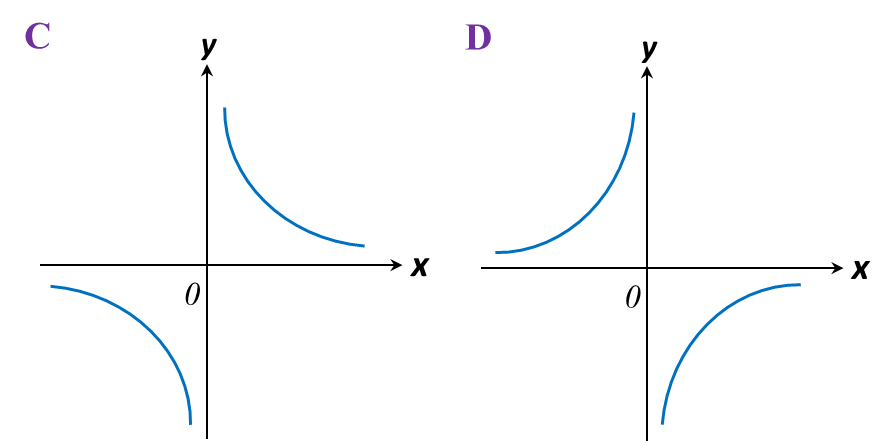# 2.4 Graph of Functions (II), SPM Paper 1 (Short Questions)

2.4 SPM Practice (Short Questions)

Question 3:
Which of the following graphs represent y = 2x3– 16?Solution:
y = 2x3 – 16
On the y-axis, x = 0.
y = 2(0)3 – 16
y = –16

The answer is C.

Question 4:
Which of the following graphs represent $y=-\frac{3}{x}?$Solution:
Highest power of the variable x is –1.
It is a reciprocal function
The answer is D.